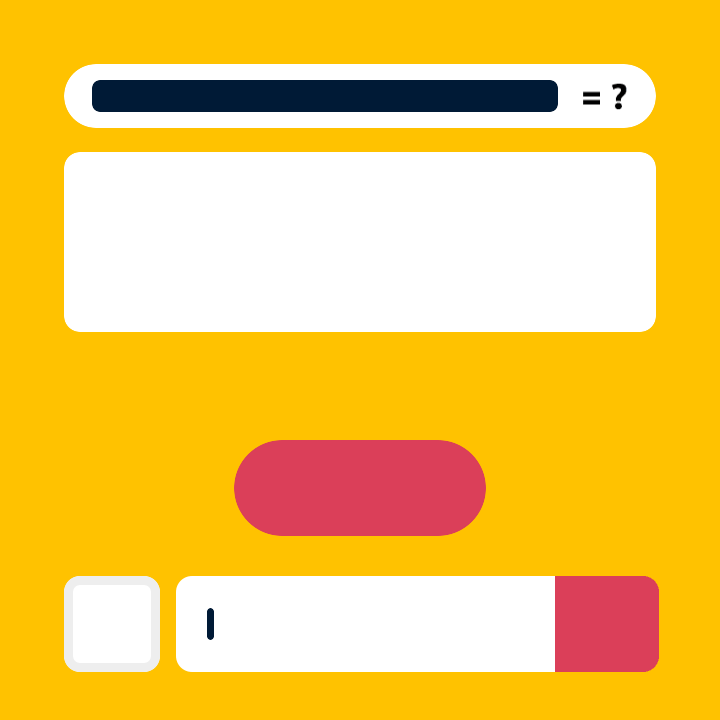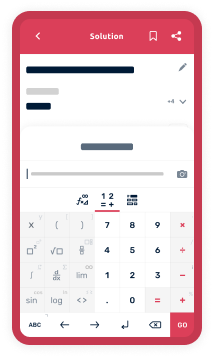\bold{\mathrm{Basic}} \bold{\alpha\beta\gamma} \bold{\mathrm{AB\Gamma}} \bold{\sin\cos} \bold{\ge\div\rightarrow} \bold{\overline{x}\space\mathbb{C}\forall} \bold{\sum\space\int\space\product} \bold{\begin{pmatrix}\square&\square\\\square&\square\end{pmatrix}} \bold{H_{2}O}
Symbolab, Making Math SimplerWord Problems
Provide step-by-step solutions to math word problemsGraphing
Plot and analyze functions and equations with detailed stepsGeometry
Solve geometry problems, proofs, and draw geometric shapes
Math Help Tailored For YouPractice
Practice and improve your math skills through interactive personalized exercises and quizzes
Also Includes
Dashboard
Track your progress with detailed performance reports and analytics
Try It OutSolution Solver
Available in the app storeMore To Explore
Calculators
Calculators and convertors for STEM, finance, fitness, construction, cooking, and more